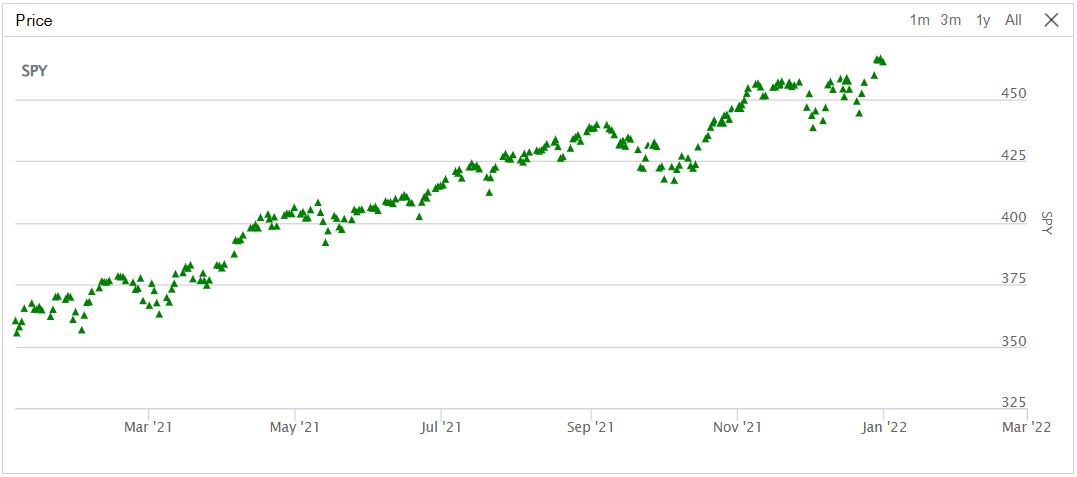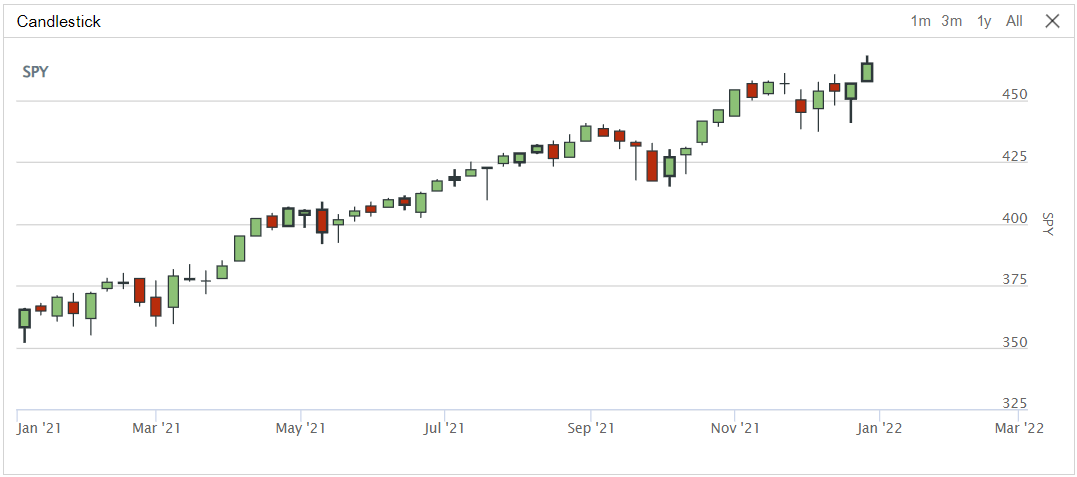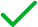# Writing Algorithms

## Charting

### Introduction

We provide a powerful charting API that you can use to build many chart types.

### Charts

Charts contain a collection of series, which display data on the chart. To add a chart to an algorithm, create a Chart object and then call the AddChart method.

var chart = new Chart("<chartName>");
AddChart(chart);
chart = Chart("<chartName>")
self.AddChart(chart)

The Chart constructor expects a name argument. The following chart names are reserved:

• Assets Sales Volume
• Exposure
• Alpha Assets
• Alpha Asset Breakdown

### Series

A chart series displays data on the chart. To add a series to a chart, create a Series object and then call the AddSeries method.

var series = new Series("<seriesName>");
chart.AddSeries(series);
series = Series("<seriesName>")
chart.AddSeries(series)

#### Arguments

There are several other headers for the Series constructor.

Series(name, type)
Series(name, type, index)
Series(name, type, index, unit)
Series(name, type, unit)
Series(name, type, unit, color)
Series(name, type, unit, color, symbol)


The following table describes the constructor arguments:

ArgumentData TypeDescription
namestringstrName of the series
typeSeriesTypeType of the series
indexintIndex position on the chart of the series
unitstringstrUnit for the series axis
colorColorColor of the series
symbolScatterMarkerSymbolSymbol for the marker in a scatter plot series

### Plot Series

To add a data point to a chart series, call the Plot method. If you haven't already created a chart and series with the names you pass to the Plot method, the chart and/or series is automatically created.

Plot("<chartName>", "<seriesName>", value);
self.Plot("<chartName>", "<seriesName>", value)

The value argument can be an integer for decimal number. If the chart is a time series, the value is added to the chart using the algorithm time as the x-coordinate.

To plot the current value of indicators, call the Plot method. The method accepts up to four indicators.

// In Initialize
var smaShort = SMA(symbol, 10);
var smaLong = SMA(symbol, 20);

// In OnData
Plot("<chartName>", smaShort, smaLong)
# In Initialize
sma_short = self.SMA(symbol, 10)
sma_long = self.SMA(symbol, 20)

# In OnData
self.Plot("<chartName>", sma_short, sma_long)

To plot all of the values of some indicators, in the Initialize method, call the PlotIndicator method. The method plots each indicator value as the indicator updates. The method accepts up to four indicators.

var symbol = AddEquity("SPY");
var smaShort = SMA(symbol, 10);
var smaLong = SMA(symbol, 20);
PlotIndicator("<chartName>", smaShort, smaLong)
symbol = self.AddEquity("SPY")
sma_short = self.SMA(symbol, 10)
sma_long = self.SMA(symbol, 20)
self.PlotIndicator("<chartName>", sma_short, sma_long)

### Plot Candlestick

To add a sample of open, high, low, and close values to a candlestick series, call the Plot method with the data points. If you haven't already created a chart and series with the names you pass to the Plot method, the chart and/or series is automatically created.

Plot("<chartName>", "<seriesName>", open, high, low, close);
self.Plot("<chartName>", "<seriesName>", open, high, low, close)

The open, high, low, and close arguments can be an integer for decimal number. If the chart is a time series, the values are added to the chart using the algorithm time as the x-coordinate.

To plot the current trade bar, call the Plot method with a TradeBar argument in the OnData method.

// In Initialize

// In OnData
var collapsed = slice.QuoteBars["EURUSD"].Collapse();   // Collapses QuoteBar into TradeBar object

Plot("<chartName2>", "<seriesName>", collapsed)
# In Initialize

# In OnData
collapsed = slice.QuoteBars["EURUSD"].Collapse()   # Collapses QuoteBar into TradeBar object
self.Plot("<chartName>", "<seriesName>", collapsed)

To plot consolidated bars, call the Plot method with a TradeBar argument in the consolidation handler.

// In Initialize
Consolidate(equity.Symbol, TimeSpan.FromMinutes(10), ConsolidationHandler);

// Define the consolidation handler
{
Plot("<chartName>", "<seriesName>", consolidatedBar)
}
# In Initialize
self.Consolidate(equity.Symbol, timedelta(minutes=10), self.consolidation_handler)

# Define the consolidation handler
def consolidation_handler(self, consolidated_bar: TradeBar) -> None:
self.Plot("<chartName>", "<seriesName>", consolidated_bar)

### Examples

The following example shows how to plot the daily closing price of SPY with a scatter plot:

public class ChartingDemoAlgorithm : QCAlgorithm
{
public override void Initialize()
{
SetStartDate(2021, 1, 1);
SetEndDate(2022, 1, 1);
var chart = new Chart("Price");
chart.AddSeries(new Series("SPY", SeriesType.Scatter, "$", Color.Green, ScatterMarkerSymbol.Triangle)); chart = new Chart("Candlestick"); AddChart(chart); chart.AddSeries(new CandlestickSeries("SPY", "$"));
}

public override void OnEndOfDay(Symbol symbol)
{
Plot("Price", "SPY", Securities[symbol].Price);
}
}
class ChartingDemoAlgorithm(QCAlgorithm):

def Initialize(self) -> None:
self.SetStartDate(2021, 1, 1)
self.SetEndDate(2022, 1, 1)
chart = Chart("Price")
chart.AddSeries(Series("SPY", SeriesType.Scatter, "$", Color.Green, ScatterMarkerSymbol.Triangle)) chart = Chart("Candlestick") self.AddChart(chart) chart.AddSeries(CandlestickSeries("SPY", "$"))

def OnEndOfDay(self, symbol: Symbol) -> None:
self.Plot("Price", "SPY", self.Securities[symbol].Price)
self.Plot("Candlestick", "SPY", self.Securities[symbol].GetLastData())To see a full example, run the CustomChartingAlgorithmCustomChartingAlgorithm.

### View Charts

The following table describes where you can access your charts, depending on how to deploy your algorithms:

LocationAlgorithm Lab AlgorithmsCLI Cloud AlgorithmsCLI Local Algorithms
Backtest results pageLive results page/backtests/read endpoint/live/read endpointReadBacktest methodReadLiveAlgorithm methodLocal JSON file in your <projectName> / backtests / <timestamp> or <projectName> / live / <timestamp> directory### Quotas

Custom charts are limited to 4,000 data points. Intensive charting requires hundreds of megabytes of data, which is too much to stream online or display in a web browser. If you exceed the quota, the following message displays:

Exceeded maximum points per chart, data skipped

You can create up to 10 custom chart series per algorithm. If you exceed the quota, your algorithm stops executing and the following message displays:

Exceeded maximum series count: Each backtest can have up to 10 series in total.

In live trading, charts are sampled every one and ten minutes. If you create 1-minute resolution custom charts, the IDE charting will downgrade the granularity and display the 10-minutes sampling after a certain amount of samples.

You can also see our Videos. You can also get in touch with us via Discord.